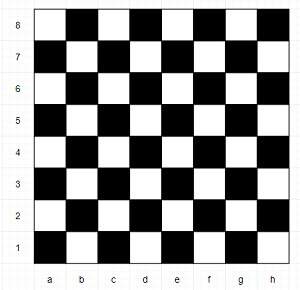# Program to determine color of a chessboard square using Python

Suppose we have a chessboard coordinate, that is a string that represents the coordinates of row and column of the chessboard. Below is a chessboard for your reference.We have to check whether given cell is white or not, if white return true, otherwise return false.

So, if the input is like coordinate = "f5", then the output will be True (See the image)

To solve this, we will follow these steps −

• if ASCII of coordinate mod 2 is same coordinate) mod 2, then

• return False

• otherwise,

• return True

Let us see the following implementation to get better understanding −

## Example

Live Demo

def solve(coordinate):
if (ord(coordinate))%2 == int(coordinate)%2:
return False
else:
return True
coordinate = "f5"
print(solve(coordinate))

## Input

"f5"

## Output

True